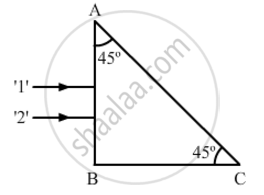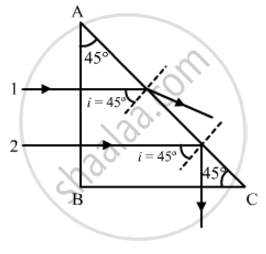# Two Monochromatic Rays of Light Are Incident Normally on the Face AB of an Isosceles Right-angled Prism ABC. the Refractive Indices of the Glass Prism for the Two Rays '1' and '2' Are Respectively 1.38 and 1.52. Trace the Path of These Rays After Entering Through the Prism. - Physics

Two monochromatic rays of light are incident normally on the face AB of an isosceles right-angled prism ABC. The refractive indices of the glass prism for the two rays '1' and '2' are respectively 1.38 and 1.52. Trace the path of these rays after entering through the prism.#### Solution

Critical angle of ray 1 is given by:

sin(c_1)=1/mu_1=1/1.38

=>c_1=sin^(-1)(1/1.38)=46.44^@

Similarly, critical angle of ray 2:

sin(c_2)=1/mu_2=1/1.52

=>c_2=sin^(-1)(1/1.52)=41.14^@

Both the rays will fall on the side AC with angle of incidence (i) equal to 45°. Critical angle for ray 1 is greater than that of i. Hence, it will emerge out from the prism as shown in the figure. Critical angle of ray 2 is less than that of i. Hence, it will get internally reflected as shown in the figure.Concept: Dispersion by a Prism
Is there an error in this question or solution?
2013-2014 (March) All India Set 3

Share